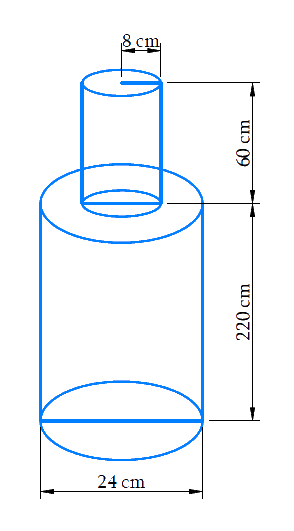# Ex.13.2 Q6 Surface Areas and Volumes Solution - NCERT Maths Class 10

Go back to  'Ex.13.2'

## Question

A solid iron pole consists of a cylinder of height $$220\,\rm{cm}$$ and base diameter $$24\,\rm{cm},$$ which is surmounted by another cylinder of height $$60\,\rm{cm}$$ and radius $$8\,\rm{cm}$$.

Find the mass of the pole, given that $$1\,\rm {cm^3}$$ of iron has approximately $$8\,\rm{g}$$ mass.

$$\left( {{\text{Use}}\,\,\pi \,{\text{ = }}\,{\text{3}}{\text{.14}}} \right)$$

## Text Solution

What is known?

A solid iron pole consisting of a cylinder of height $$220\,\rm{cm}$$ and base diameter  $$24\,\rm {cm}$$  which is surmounted by another cylinder of height  $$60\,\rm {cm}$$ and radius  $$8\,\rm {cm}$$

Mass of $$1\;\rm{cm}^3$$ iron $$= 8\rm\,{g}$$

What is unknown?

The mass of the solid iron pole

Reasoning:

Draw the figure to visualize the iron poleVisually it’s clear that

Volume of the solid iron pole $$=$$ volume of larger cylinder $$+$$ volume of smaller cylinder

Mass of iron in the pole  $$=8\,\rm{g}$$ $$\;\times\;$$volume of the solid iron pole in $${\text{c}}{{\text{m}}^3}$$

We will find the volume of the solid by using formula;

Volume of the cylinder$$= \pi {r^2}h$$

where $$r$$ and $$h$$ are the radius and height of the cylinder respectively.

Steps:

Radius of larger cylinder,\begin{align}R = \frac{{24cm}}{2} = 12cm\end{align}

Height of larger cylinder,$$H = 220cm$$

Radius of smaller cylinder,$$r = 8cm$$

Height of smaller cylinder,$$h = 60cm$$

Volume of the solid iron pole $$=$$ volume of larger cylinder $$+$$ volume of smaller cylinder

\begin{align}&= \pi {R^2}H + \pi {r^2}h\\&= \pi \left( {12cm \times 12cm \times 220cm + 8cm \times 8cm \times 60cm} \right)\\&= 3.14 \times \left( {31680c{m^3} + 3840c{m^3}} \right)\\&= 3.14 \times 35520c{m^3}\\&= 111532.8c{m^3}\end{align}

Mass of $$1cm^3$$ iron is $$8g$$

Mass of iron in the pole $$= 8g ×$$ volume of the solid iron pole in $$cm^3$$

\begin{align}&= 8g \times 111532.8\\&= 892262.4g\\&= \frac{{892262.4}}{{1000}}kg\\&= 892.2624kg\end{align}

Mass of iron in the pole is $$892.26\,\rm{ kg}$$

Learn from the best math teachers and top your exams

• Live one on one classroom and doubt clearing
• Practice worksheets in and after class for conceptual clarity
• Personalized curriculum to keep up with school